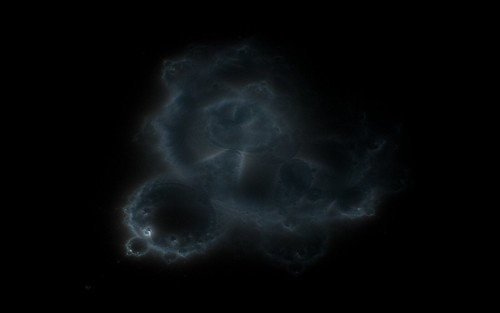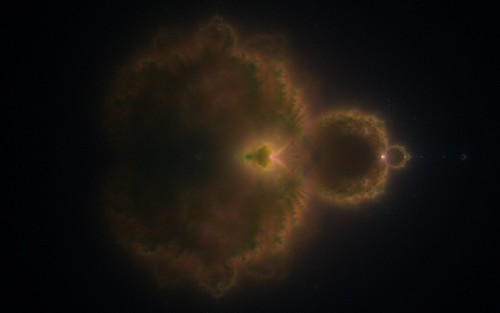Note: This is an older post and the gravity simulation code has since been updated. See here for the latest.

I recently revisited the 3D Gravity simulation code in Visions Of Chaos. Adding a few new features really helped improve the resulting outcomes.

First is changing to 2nd order Euler integration. This gives a much more accurate result in the force between objects calculations. Using 4th order Runge-Kutta would be the ideal and is planned for the future.

Second is adding a setting for minimum distance that objects interact. If you use the default Newtonian gravity formulas for 2 objects and they are too close to each other then this will usually lead to one or both of the objects being flung off out of the simulation area at an enormous speed. Even though this is the correct accurate result it generally leads to all objects being flung out of the screen within the first hundred steps or so. Including a minimal distance means that force calculations between any objects closer than a small value are ignored. A good factor seems to be around 1% or so of the world size.

Third was adding trails tracing to the particles. After implementing this with the Boids code it was simple enough to use it with the 3D Gravity too.

Here is a movie showing the new results. First part is a circular cloud of particles and the second part is a top down disc of rotating objects.

Jason.

# Buddhabrot Fractals

Overview

Buddhabrots are a different way of visualizing the Mandelbrot Set. They fall into the general catagory of an “orbit density” plot. The Buddhabrot was discovered by Melinda Green and named Buddhabrot because if you rotate the image 90 degrees clockwise it apparently resembles a sitting Buddha. Just another case of Pareidolia if you ask me.

Images based on orbit density plots had been explored before (see these examples from Linas Vepstas), but the Buddhabrot method was the first to ignore orbits that start within the Mandelbrot Set and only plot points outside the set. This unique discovery led to the Buddhabrot images.To create a Buddhabrot image, you track the orbits of random starting points on the complex plane that are outside the Mandelbrot Set and count the number of times each on screen pixel is hit. These pixel hits accumulate and create the Buddhabrot image.

Calculating the image

Here is the basic idea

1. Pick a random starting point for complex C. A good range is between -0.5 and +2 for the real axis and -1.3 to +1.3 on the imaginary axis.
2. Iterate z=z^2+c in the usual Mandelbrot way until the iterations reach a specified maximum iterations limit or the magintude of z is greater than a bailout value.
3. If z did exceed the bailout, it is a point not in the set, so reiterate the initial random point and each pixel it hits on screen you increase the hit count.
4. Every so often use the hitcount buffer to build the image you plot to the screen.
5. Goto 1 as long as it takes until a smooth image is rendered.

Speedups

The above method will work and if you have a lot of patience you will slowly start to see a Buddhabrot image appear, but there are many ways to speed up the process of rendering Buddhabrots. Here are a few I found and implemented in the latest version of Visions Of Chaos.

1. When looking for a random starting point for each orbit, the main cardioid and smaller 2nd period bulb areas of the Mandelbrot set can be easily detected and instantly skipped (saving the time of waiting for the z=z^2+c iterations to reach the maximum iteration value).

``````
function SkipCardioidAndCircle(a,b:double):boolean;
var cr,ci:double;
begin
//circle to the left
if (((a+1)*(a+1)+b*b)=-0.75) then
begin
cr:=a*a+b*b;
ci:=sqrt(cr-a/2+0.0625);
if ((16*cr*ci)<(5*ci-4*a+1)) then
begin
SkipCardioidAndCircle:=true;
exit;
end;
end;
SkipCardioidAndCircle:=false;
end;
```
```

2. Use a good random number generator. The default random number generators in most compilers have a fairly low period of values before they start repeating the same numbers again. For the Buddhabrot you need a random number function that can return a huge number of random values before they start repeating. A good (and fast) generator I use is the Mersenne Twister.

3. Take advantage of the symmetry of the Mandelbrot Set. Each time a point on the orbit is found, it has a mirror point around the imaginary axis. Checking for these is minimal code and if you are rendering the whole image or any area around the imaginary Y axis then this gives a nice 2x speedup (and also gives the renders a symmetric look).

4. Do not reiterate the initial point if a valid orbit is found. Create an array or list and store the points the orbit goes through while testing. If you find it is a valid orbit you can use the array of points to update the hitcount buffer without the overhead of going through the iteration again.

5. Periodocity checking. As you iterate each point do a quick check to see the point is going through the same set of points. Basics of this is you keep track of an oldz complex value. If the new z during the z=z^2+c matches the old one then you know you can stop iterating. You can set the oldz to z after each iteration but this only checks for single period orbits. The better method is Brent’s Method. Any time the iteration is a power of 2 you set the oldz to z.

Using the above simple speedups gave me a massive improvement in performance. Without those I had a long slow wait until the familiar buddhabrot image appeared. With the speedups leads to millions of pixles per second which gets the general image appearing in no time.

There are of course many more ways to speedup the code using tricks specific to your compiler of choice, but those 5 Buddhabrot specific methods will give orders of magnitude increases in speed with minimal effort.

SQRT Coloring

Once you have your buffer to keep track of how many times each pixel has been hit, you need a way of converting these hit values into an image.

The naive approach is to simply find the maximum hitcount across the image and get a color value by colval=hitcount/maxcount*255 and setting the pixel color to RGB(colval,colval,colval) or using the colval to map a color index in a 256 color palette. This works, but gives a result that hides a lot of the finer details.

A better approach is to use sqrt scaling. Calculate the colval by colval=trunc(255*sqrt(hitcount)/sqrt(maxcount)). This leads to images that are not washed out in the high end of the hitcount and also not too pixelated in the low end.To add a gamma value to sqrt coloring you can use colval=trunc(255*power(hitcount/maxcount,1/gamma)) with a gamma value between 1 and 10. Gamma 2 is equal to the usual sqrt coloring. Thanks to Stefan (see comments) for this tip.

Nebulabrot Coloring

This coloring idea was inspired from the way NASA colors their Hubble images. Each of the RGB channels in an image is collected using different wavelengths and then when combined they make a multi-color image.

The same technique can be applied to Buddhabrot images. You need 3 arrays that keep track of the hitcount for the red, green and blue channels. Each RGB value has a starting and ending iteration range. For example, you iterate for a maximum of 1000 iterations over the image and red is set to the range of between 0 and 50 iterations, green is set to range of 50 to 200 iterations and blue is set to the range of 200 to 1000 iterations. If the iteration count of a certain pixel is 250 then only the blue hitcount buffer is updated. The buffers are then individually sqrt scaled and then combined into the final pixel RGB color. This gives images like the following;Metropolis-Hastings

The main problem with using the Buddhabrot method to render images is when you zoom into them. The initial random points selected for each new orbit need to remain in the range between -0.5 and +2 for the real axis and -1.3 to +1.3 on the imaginary axis to give an accurate image without artifacts. But, the more you zoom into an image, the fewer of these random initial points will lead to an orbit that passes through the “visible area” you are zoomed into. Even at moderate zoom levels this leads to a few pixels hit per second and unless you are willing to leave your PC churning away for a week you are not going to get reasonable quality results from zoomed in images.

Alexander Boswell explains how to use the Metropolis-Hastings method to speed up zoomed in Buddhabrots on this page. This is a superb way to speed up zoomed in Buddhabrots.

The basic idea is that you look for good orbits and then try slight mutations of them. The reason being is that if one random starting point of an orbit goes through your visible zoomed in area, then other points close to it will too.

Here are few moderately zoomed in Buddhabrot images. These took minutes rather than weeks to render.

This first one is at 519 times magnification.And this one is at 2,427 times magnification.